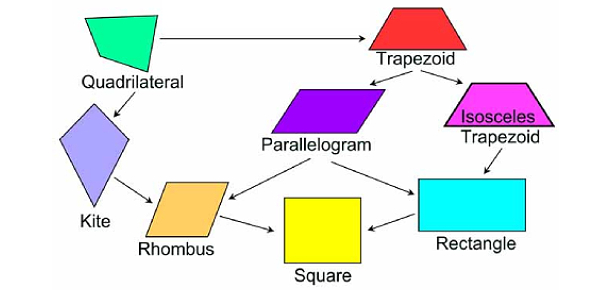# Quadrilateral And Its Types Quiz

10 Questions | Total Attempts: 79SettingsA quadrilateral is a polygon that has exactly four sides. Here is an informative quiz about Quadrilaterals, that will help in the betterment of your geometric understanding. The quiz will help you learn more about all the 4 sided mathematical figures to understand the concepts of maths, trigonometry, and other similar topics properly.

• 1.
All of the following options are a type of quadrilateral except,
• A.

Rhombus

• B.

Square

• C.

Triangle

• D.

Trapezoid

• 2.
Which one of the following are a quadrilateral with 4 equal sides and right angles.
• A.

Trapezoid

• B.

Square

• C.

Rectangle

• D.

Parallelogram

• 3.
A quadrilateral with 2 pairs of parallel sides and 4 right angles.
• A.

Trapezoid

• B.

Square

• C.

Rectangle

• D.

Rhombus

• 4.
Which of the set of figure shows a group of quadrilateral?
• A.

Option 1

• B.

Option 2

• C.

Option 3

• D.

Option 4

• 5.
• A.

A quadrilateral has 4 sides, 4 angles and 4 vertices.

• B.

B. The sum of all the interior angles of a quadrilateral is 360°.

• C.

C. A quadrilateral can be classified into Parallelograms, Squares, Rectangles, rhombuses and trapezoids.

• D.

D. quadrilateral is a two-dimensional shape which has 3 sides.

• 6.
• 7.
A ____ quadrilateral features 2 pairs of adjacent sides that are of equal length (much like a ___ you can fly.)
• 8.
Quadrilaterals with 2 sets of parallel side are called ________?
• A.

Square

• B.

Rectangle

• C.

Rhombus

• D.

Parallelogram

• 9.
The perimeter of a square is __ times the length of one side.
• A.

4

• B.

8

• C.

2

• D.

16

• 10.
A quadrilateral with 4 right angles is a
• A.

Square

• B.

Rectangle

• C.

All of the above

• D.

None

Related TopicsBack to top